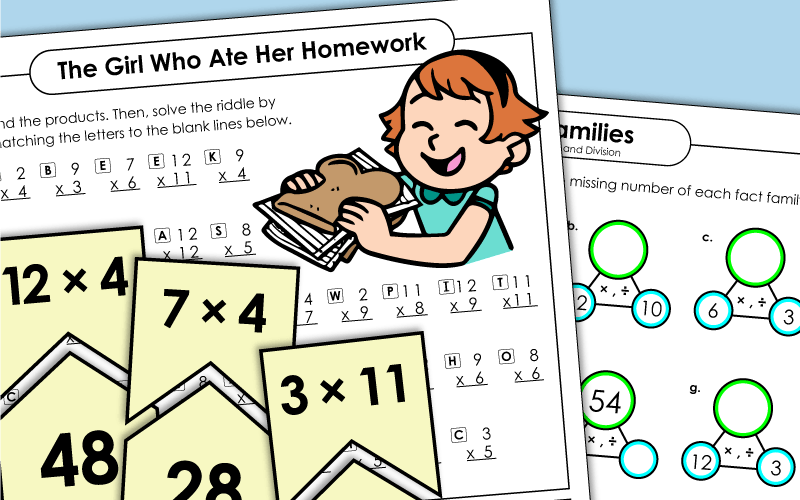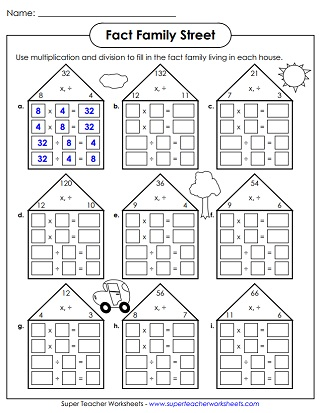# Basic Multiplication Facts (Through 12s)

Basic multiplication printables for teaching basic facts through 12x12. Print games, quizzes, mystery picture worksheets, flashcards, and more.

If you're teaching basic facts between 0 and 10 only, you may want to jump to our Multiplication 0-10 page.## Games

Practice basic multiplication from 0-12 with this printable puzzle activity.
Lay all of the cards on the table face-down. Players take turns trying to find multiplication facts and their matches.
Cut and glue to create a board game with question cards. Travel to the moon as you answer basic multiplication facts.
When playing this game, students roll a die. The number they roll corresponds to a column of math facts on the game board. Students read and answer the facts as quickly as possible.
Circle each multiplication fact you find on this number hunt. There are 20 facts to find.
Print the 36 cards in this set on card stock. Then cut them apart. Your students can match the facts, array graphics, and answers/products. You can also play a memory-match game with this set of cards.
This is a whole-class chain reaction game for reviewing basic multiplication facts up to 12.

## Flashcards

Copy these flashcards on card stock and let your students cut them out and practice with them.
Copy these flashcards (large) on card stock and let your students cut them out and practice with them.
Practice basic multiplication facts with these triangular fact family cards.

## Worksheets (0-12s)

Why did the girl eat her homework? To find the funny answer to this riddle, students will need to solve 41 multiplication facts.
First cut out a multiplication fact and glue it in the center of the page. Then write a repeated addition problem, create an array, and draw a grouping illustration.
Scissors and a glue stick are needed for this multiplication worksheet. Cut out the number boxes on the page and glue them to the appropriate box.
Write the answers to the multiplication facts, then write X or O over the corresponding numbers on the tic-tac-toe board.
There are twenty multiplication facts on this page. Each one has a missing factor. Write the missing number for each problem. Up to 12 times 12.
Complete the multiplication number bonds. Includes facts up to 12s.
Solve these basic 1-12 multiplication problems and then use the solutions to find the right color for each space to reveal school supplies on a desk.
The multiplication problems on this page are from 1-12. Solve them and use the color key to determine how it is colored to reveal sea shells on the beach.
Write the answers to these multiplication facts on this printable caterpillar worksheet. These multiplication problems all have factors of 12 or less.
Decode the symbols using the cypher to reveal the mystery numbers. Then multiply the factors together and write the products.
Insert factors that will complete the puzzle grid to make the given products.

This worksheet shows fruit divided into groups. Students use repeated addition to find the totals. Then write each as a multiplication fact.

## Multiplication Tables(Multiplication Charts)

This is a complete, full-page multiplication chart with all boxes filled in.
Complete these mini-multiplication tables by filling in the missing products. This sheet has butterfly-themed graphics.
Complete the multiplication tables by filling in the missing factors and products. This worksheet has winter sports-themed illustrations.
This PDF has a blank multiplication table (up to 12) that students can fill in.
This chart is partly filled in, but student have to fill in the remaining boxes.
This multiplication table has number 0 through 12 on the X and Y axis, but the numbers aren't in order.
This document has four miniature multiplication tables on a single page. Cut them out for your kids to use as a reference tool.
Here is a different style chart. It has all facts listed. Answers not included.
This is just like the chart above, but this version does show products.

## Basic MultiplicationWorksheet Generator

Basic Multiplication
Worksheet Generator

Make your own basic multiplication worksheets. You choose the range for the first and second factor. This generator allows you to create worksheets with 25 or 50 problems.

## Fact Families

Students are presented with a number family. They have to write two division and two multiplication facts for each.
Write the missing number for each factor-factor-product box.
Complete each number family by writing the missing value in the empty circles.

## Timed Quizzes

Here's a multiplication quiz for your students.  Facts include 0s, 1s, 2s, 3s, 4s, 5s, 6s, 7s, 8s, 9s, 10s, 11s, and 12s.
This fifty question quiz has facts up to twelve times twelve.
This PDF contains the third version of the 0 through 12 multiplication quiz.

## Multiplication Drills

This multiplication drill sheet has pictures of funny ducks. There are 39 problems in all.
Need more practice on multiplying basic facts? Here is another sheet of multiplication drills.

## Origami Fortune Tellers(aka Cootie Catchers)

Color, then cut, then fold the fortune teller. Includes eight basic facts with elevens. (Up to 11x12)
Learn those tricky twelves with this paper-folding game. (Up to 12x12)
Basic Multiplication (0-10)

If you're only teaching basic facts up to 10, try the worksheets and games on this page.

More Basic Multiplication

This index page will link you to many more multiplication sections on our site.

Fact Family Worksheets

This page has fact families and number bonds for teaching students about the relationships between multiplication and division.

Properties of Multiplication

Learn about the associative, distributive, and commutative properties with these printables.

Multi-Digit Multiplication

More advanced multiplication activities, with multiple digits can be found in this section of the site.

## Sample Worksheet ImagesMy Account
Site Information